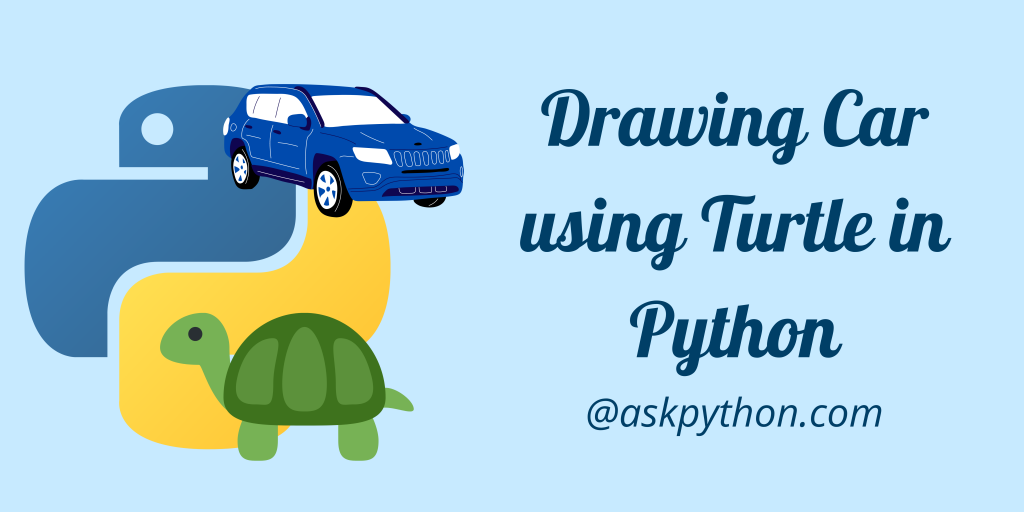# Drawing Car using Turtle in PythonHey coder! In this tutorial, I will teach you how to draw your own car with the help of the python turtle library. If you are unaware of the `turtle` module, check out the tutorial here.

## Steps to Draw a Car Using Python Turtle

You need to import the turtle library that comes with Python and there is no need to do any additional installing.

```import turtle
```

The next step involves creating an application screen to draw the car. We can name the window anything we wish to. For this tutorial, we have kept the name of the screen as `car_scr`.

The code below does the creation and customization of the screen including the color of the screen and pen.

```import turtle
car_scr = turtle
car_scr.color('black')
car_scr.Screen().bgcolor("#ABEBC6")
```

Now let’s create a function that will draw the car for us. The car has a number of different parts such as the upper body, wheels, and windows. We will draw each of them separately one after another.

Inside the function `Draw_Car`, we need to give the starting coordinates to the function as a parameter. Then the `goto` function takes the pointer to the position we have passed to the function.

You might be familiar with the functions used in the Draw_Car function. I am going to mention how each works below:

1. `penup` & `pendown` – Control when to draw and when not to.
2. `fillcolor``begin_fill` and `end_fill` – Control the colors for a particular figure
3. `forward` & `backward` and `left` & `right` – Helps to draw on the screen in a particular direction or angle.

### Creating the Base of the Car Using Python Turtle

Let us first draw the upper body of the car using the code below. Look how great the output turns out to be.

```def Draw_Car(i,j):
# Drawing the Upper Body
car_scr.fillcolor("#2980B9")
car_scr.penup()
car_scr.goto(i,j)
car_scr.pendown()
car_scr.begin_fill()
car_scr.forward(370)
car_scr.left(90)
car_scr.forward(50)
car_scr.left(90)
car_scr.forward(370)
car_scr.left(90)
car_scr.forward(50)
car_scr.end_fill()
```

### Draw Car Windows

We will call the function and pass the starting x and y values. Let’s call the function for `Draw_Car(-200,0)`. Now we will be drawing the windows using a very similar code as shown above. Have a look at the code below.

```def Draw_Car(i,j):
# Drawing the Upper Body
car_scr.fillcolor("#2980B9")
car_scr.penup()
car_scr.goto(i,j)
car_scr.pendown()
car_scr.begin_fill()
car_scr.forward(370)
car_scr.left(90)
car_scr.forward(50)
car_scr.left(90)
car_scr.forward(370)
car_scr.left(90)
car_scr.forward(50)
car_scr.end_fill()

#Draw the Windows
car_scr.fillcolor("#D5DBDB")
car_scr.penup()
car_scr.goto(i+100, 50)
car_scr.pendown()
car_scr.begin_fill()
car_scr.forward(70)
car_scr.forward(100)
car_scr.forward(70)
car_scr.end_fill()
car_scr.penup()
car_scr.goto(i+200, 50)
car_scr.pendown()
car_scr.forward(49.50)
```

When we execute this code, we get the following screen as output. Pretty amazing right?!

### Adding Car Wheels Using Turtle

Lastly, we need to add wheels to the car in a similar fashion. Just look at the complete function below. In the end, we are going to hide the turtle pointer to see a clean car image!

```def Draw_Car(i,j):
# Drawing the Upper Body
car_scr.fillcolor("#2980B9")
car_scr.penup()
car_scr.goto(i,j)
car_scr.pendown()
car_scr.begin_fill()
car_scr.forward(370)
car_scr.left(90)
car_scr.forward(50)
car_scr.left(90)
car_scr.forward(370)
car_scr.left(90)
car_scr.forward(50)
car_scr.end_fill()

#Draw the Windows
car_scr.fillcolor("#D5DBDB")
car_scr.penup()
car_scr.goto(i+100, 50)
car_scr.pendown()
car_scr.begin_fill()
car_scr.forward(70)
car_scr.forward(100)
car_scr.forward(70)
car_scr.end_fill()
car_scr.penup()
car_scr.goto(i+200, 50)
car_scr.pendown()
car_scr.forward(49.50)

# Draw the two wheels
car_scr.penup()
car_scr.goto(i+100, -10-j)
car_scr.pendown()
car_scr.color('black')
car_scr.fillcolor('black')
car_scr.begin_fill()
car_scr.circle(20)
car_scr.end_fill()
car_scr.penup()
car_scr.goto(i+300, -10-j)
car_scr.pendown()
car_scr.color('black')
car_scr.fillcolor('black')
car_scr.begin_fill()
car_scr.circle(20)
car_scr.end_fill()

car_scr.hideturtle()
```

### Run Python Turtle

Let’s draw the car on the screen using the code below. And after we are done drawing the car we will close the application screen with the help of the `done` function.

```Draw_Car(-200,0)
car_scr.done()
```

## Complete Python Turtle Code To Draw A Car

```import turtle
car_scr = turtle
car_scr.color('black')
car_scr.Screen().bgcolor("#ABEBC6")

def Draw_Car(i,j):
# Drawing the Upper Body
car_scr.fillcolor("#2980B9")
car_scr.penup()
car_scr.goto(i,j)
car_scr.pendown()
car_scr.begin_fill()
car_scr.forward(370)
car_scr.left(90)
car_scr.forward(50)
car_scr.left(90)
car_scr.forward(370)
car_scr.left(90)
car_scr.forward(50)
car_scr.end_fill()

#Draw the Windows
car_scr.fillcolor("#D5DBDB")
car_scr.penup()
car_scr.goto(i+100, 50)
car_scr.pendown()
car_scr.begin_fill()
car_scr.forward(70)
car_scr.forward(100)
car_scr.forward(70)
car_scr.end_fill()
car_scr.penup()
car_scr.goto(i+200, 50)
car_scr.pendown()
car_scr.forward(49.50)

# Draw the two wheels
car_scr.penup()
car_scr.goto(i+100, -10-j)
car_scr.pendown()
car_scr.color('black')
car_scr.fillcolor('black')
car_scr.begin_fill()
car_scr.circle(20)
car_scr.end_fill()
car_scr.penup()
car_scr.goto(i+300, -10-j)
car_scr.pendown()
car_scr.color('black')
car_scr.fillcolor('black')
car_scr.begin_fill()
car_scr.circle(20)
car_scr.end_fill()

Draw_Car(-200,0)

car_scr.done()
```

When we execute the code above, a new screen comes on the system screen and the car begins to draw on the screen in the application.

## Conclusion

Congratulations! Now you know how to draw a car on the screen using the Turtle library in the Python programming language. Thank you for reading!

If you liked this tutorial, I would recommend you to go through the following tutorials as well: